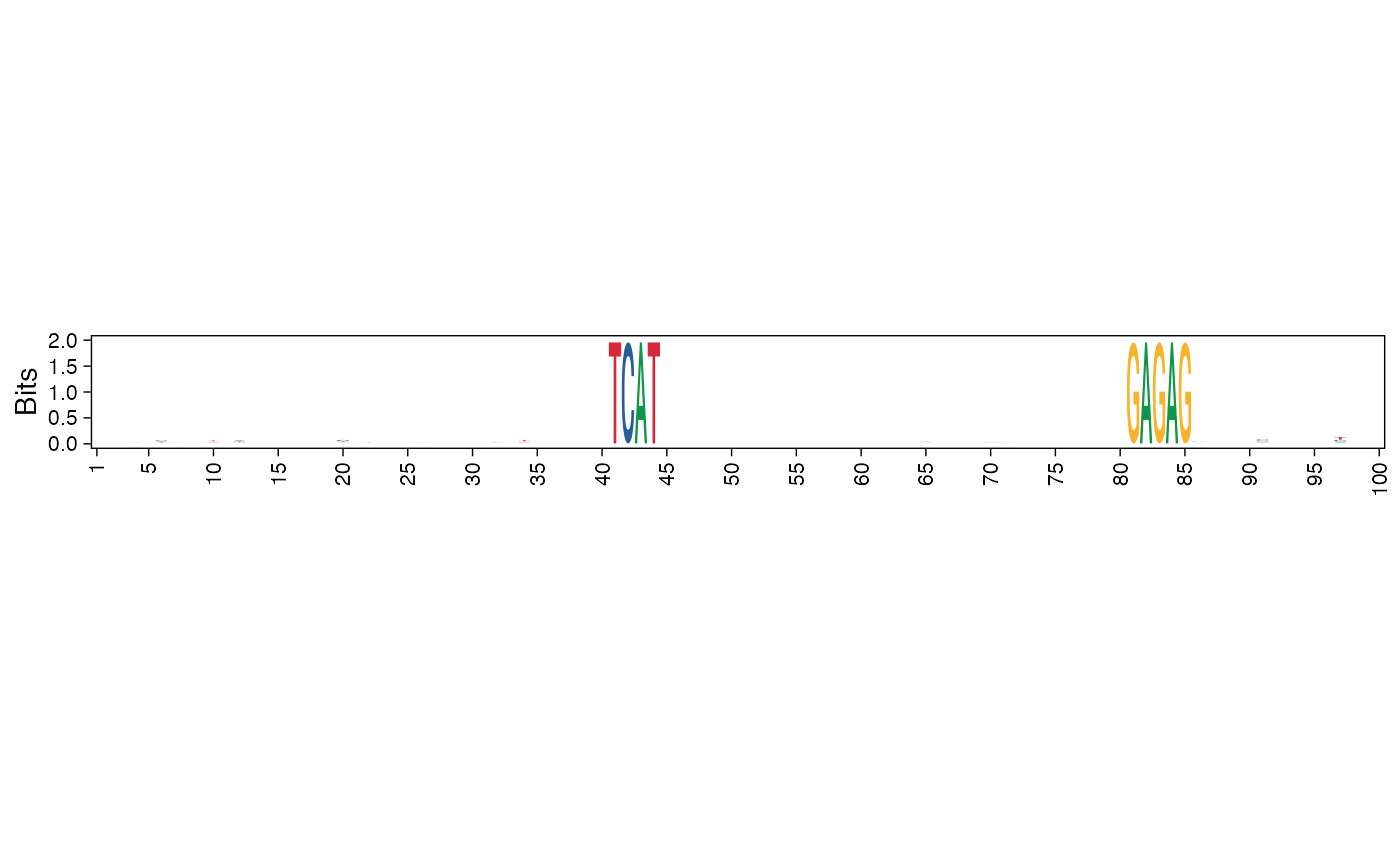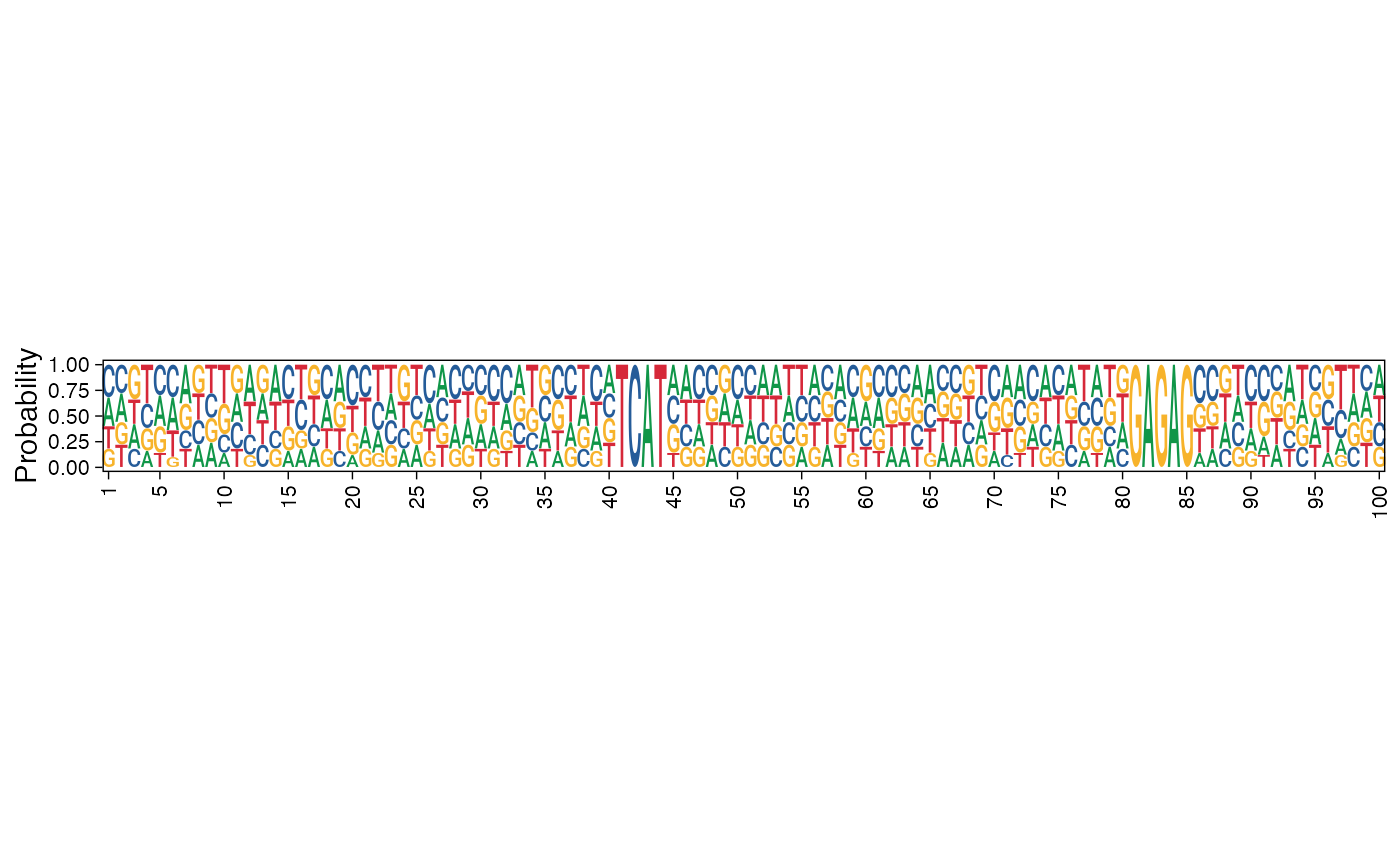A wrapper to ggseqlogo plotting. Given a collection of sequences, this function plots the sequence logo.

plot_ggseqlogo_of_seqs(
seqs,
pos_lab = NULL,
xt_freq = 5,
method = "bits",
title = NULL,
bits_yax = "full",
fixed_coord = FALSE
)

## Arguments

seqs

Collection of sequences as a DNAStringSet object.

pos_lab

Labels for sequence positions, should be of same length as that of the sequences. Default value is NULL, when the positions are labeled from 1 to the length of the sequences.

xt_freq

Specify the frequency of the x-axis ticks.

method

Specify either 'bits' for information content or 'prob' for probability.

title

The title for the plot. Deafult is NULL.

bits_yax

Specify 'full' if the information content y-axis limits should be 0-2 or 'auto' for a suitable limit. The 'auto' setting adjusts the y-axis limits according to the maximum information content of the sequence logo. Default is 'full'.

fixed_coord

Specify TRUE if the aspect ratio of the plot should be fixed, FALSE otherwise. Default is TRUE. When method argument is set to 'bits', ratio is 4, when 'prob', ratio is 6.

## Value

A sequence logo plot of the given DNA sequences.

plot_arch_for_clusters for obtaining multiple sequence logo plots as a list.

## Examples

res <- readRDS(system.file("extdata", "example_seqArchRresult.rds",
package = "seqArchR", mustWork = TRUE))

# Default, using information content on y-axis
pl <- plot_ggseqlogo_of_seqs(seqs = seqs_str(res, iter=1, cl=3),
pos_lab = seq_len(100), title = NULL,
fixed_coord = TRUE)
#> Scale for x is already present.
#> Adding another scale for x, which will replace the existing scale.
#> replace the existing one.
pl# Using probability instead of information content
pl <- plot_ggseqlogo_of_seqs(seqs = seqs_str(res, iter=1, cl=3),
pos_lab = seq_len(100), title = "",
method = "prob", fixed_coord = TRUE)
#> Scale for x is already present.
#> Adding another scale for x, which will replace the existing scale.